# RS Aggarwal Solutions for Class 7 Maths Chapter 5 Exponents

RS Aggarwal Solutions for Class 7 Maths Chapter 5 – Exponents are available here. Our professional tutors formulate these exercises to help you with your exam preparation to attain good marks in Maths. Students aiming to score good marks in Maths practise RS Aggarwal Solutions, which help them to gain more knowledge. This book is one of the top materials when it comes to providing a question bank to practice.

The foremost objective is to help students understand and crack these problems. We at BYJU’S have prepared the RS Aggarwal Solutions for Class 7 Maths Chapter 5 wherein problems were solved step by step with complete descriptions. Download pdf of Class 7 Chapter 5 in their respective links.

## Download PDF of RS Aggarwal Solutions for Class 7 Maths Chapter 5 – Exponents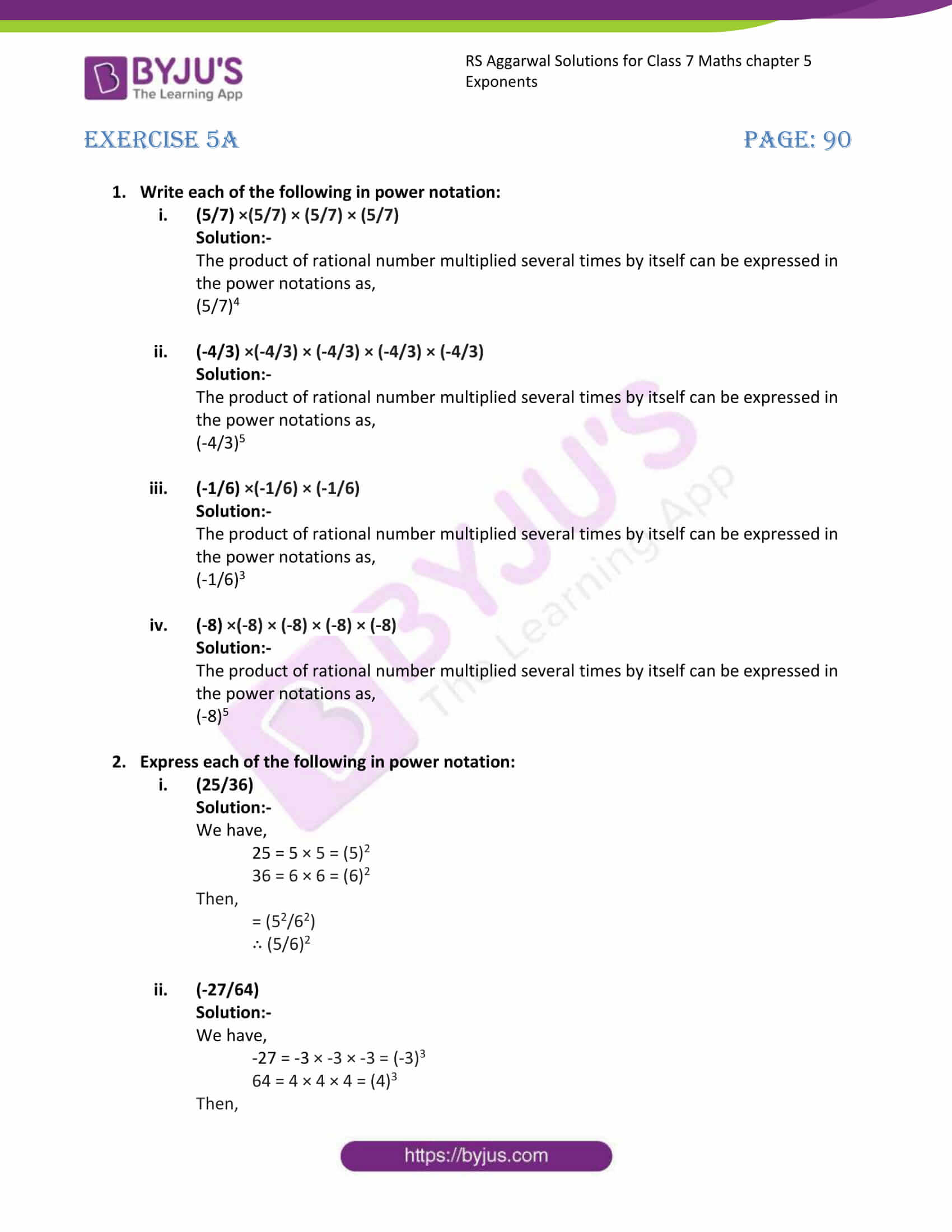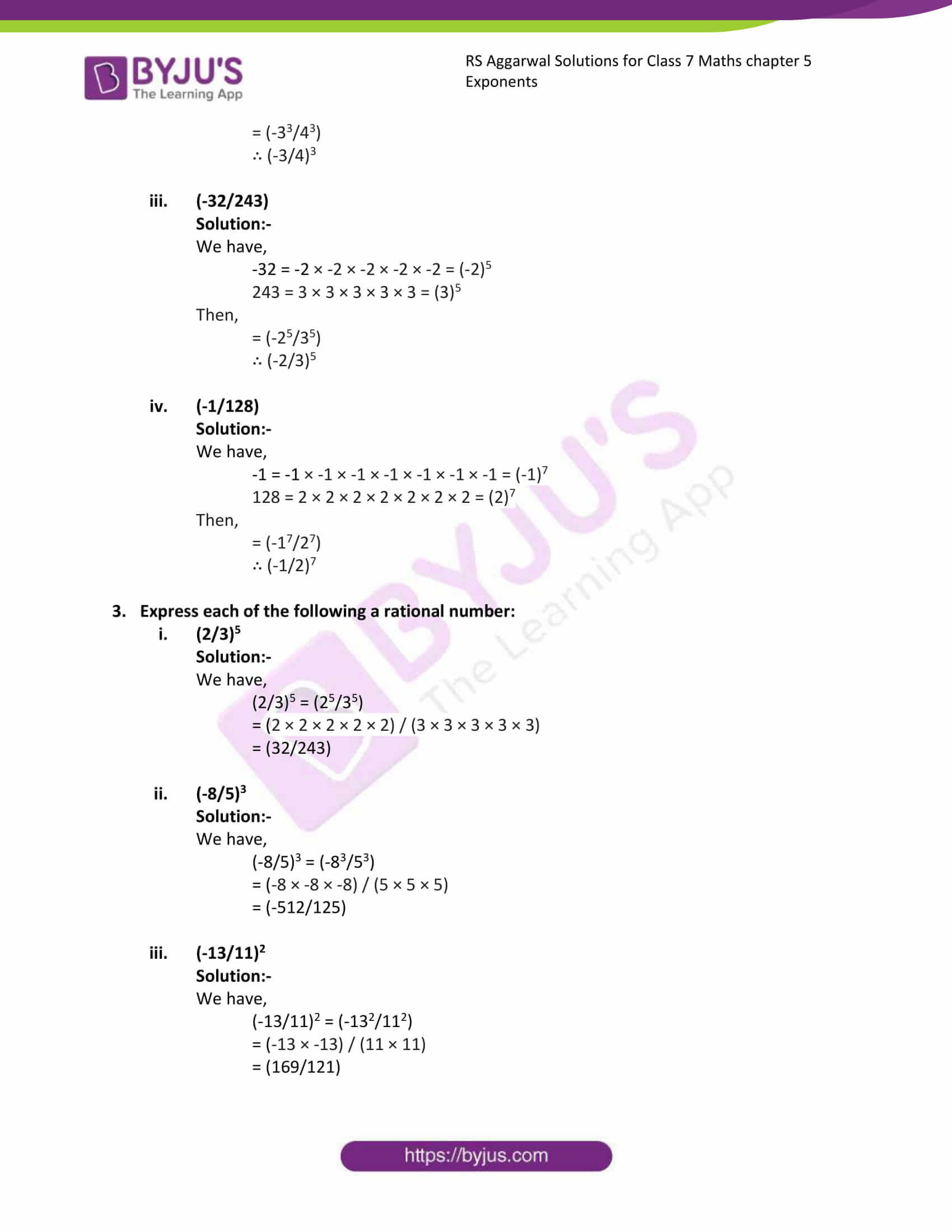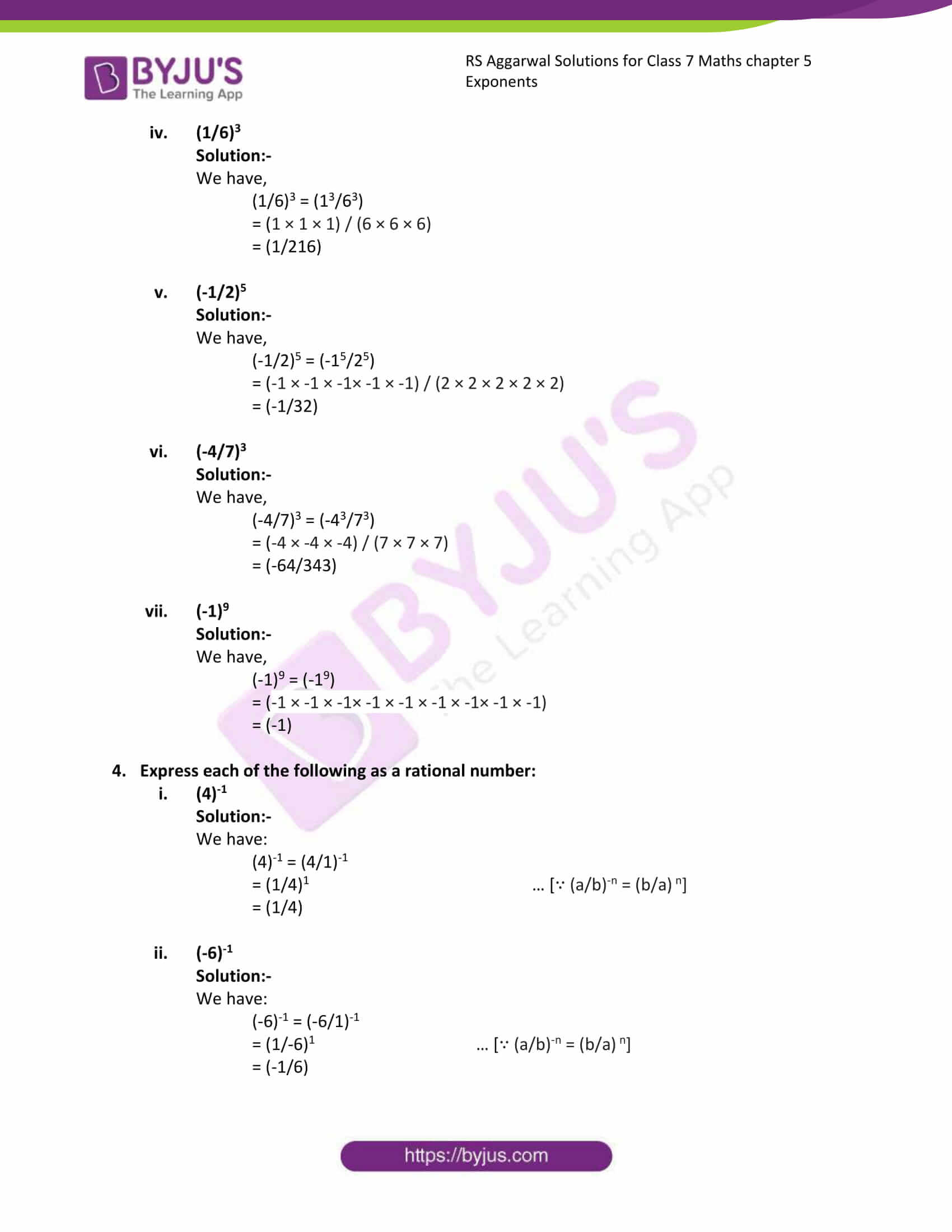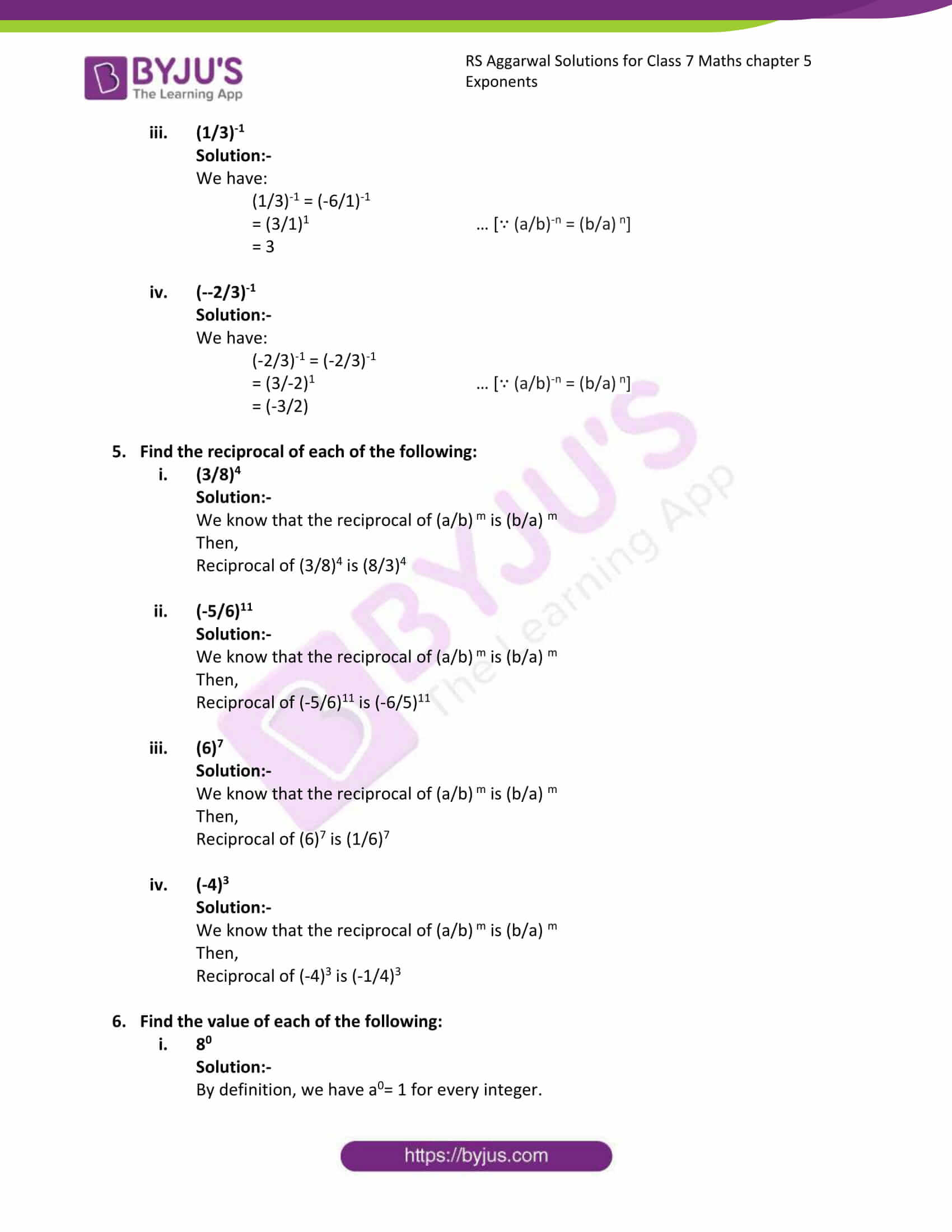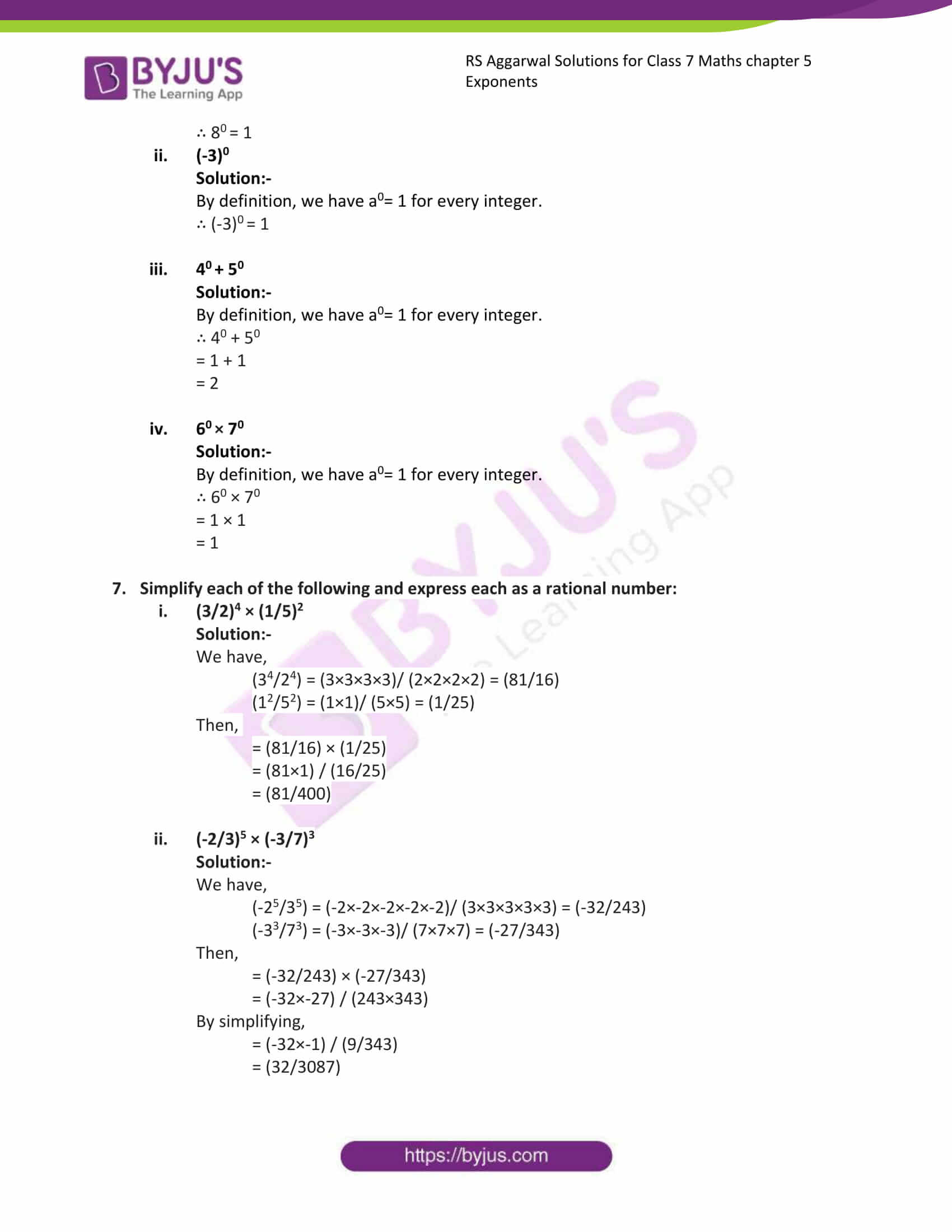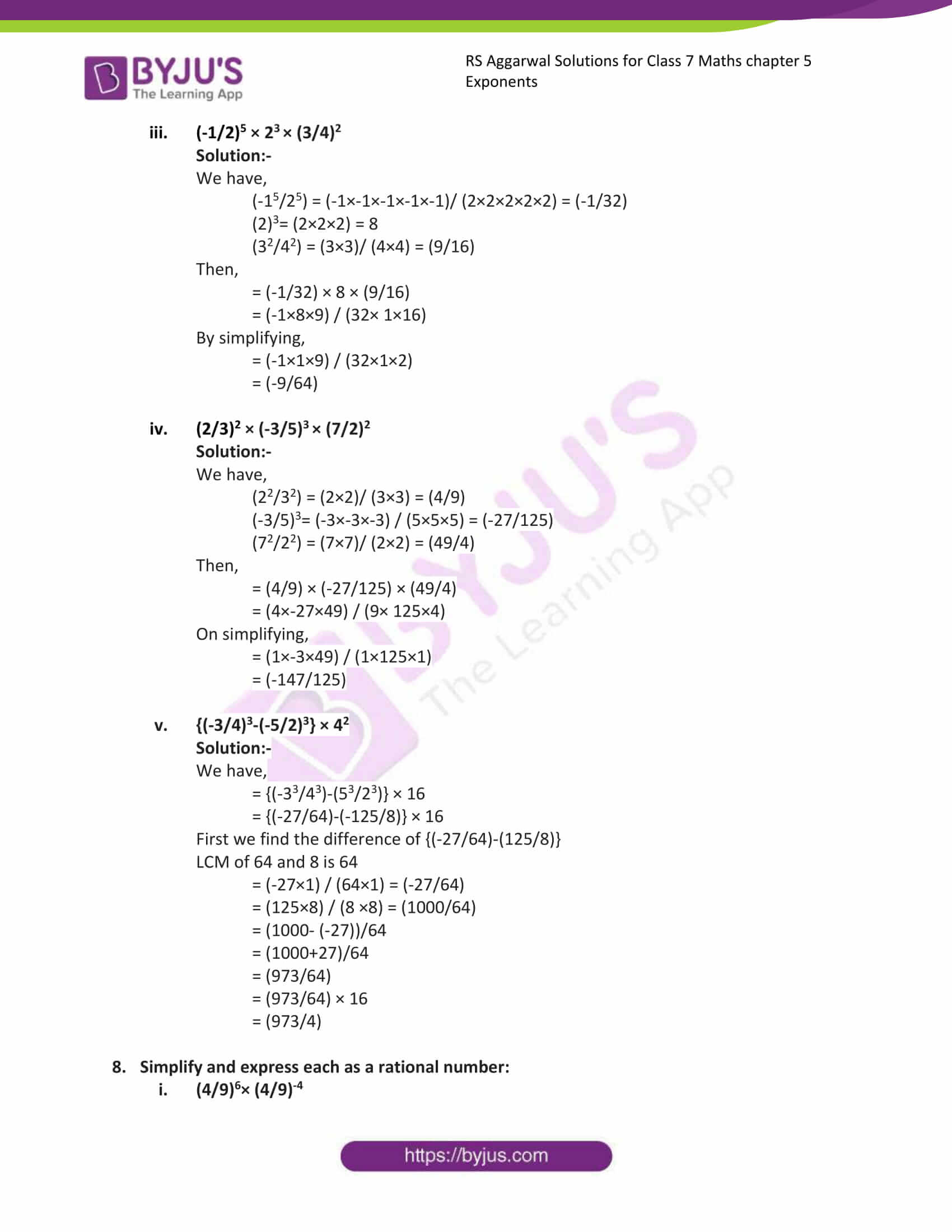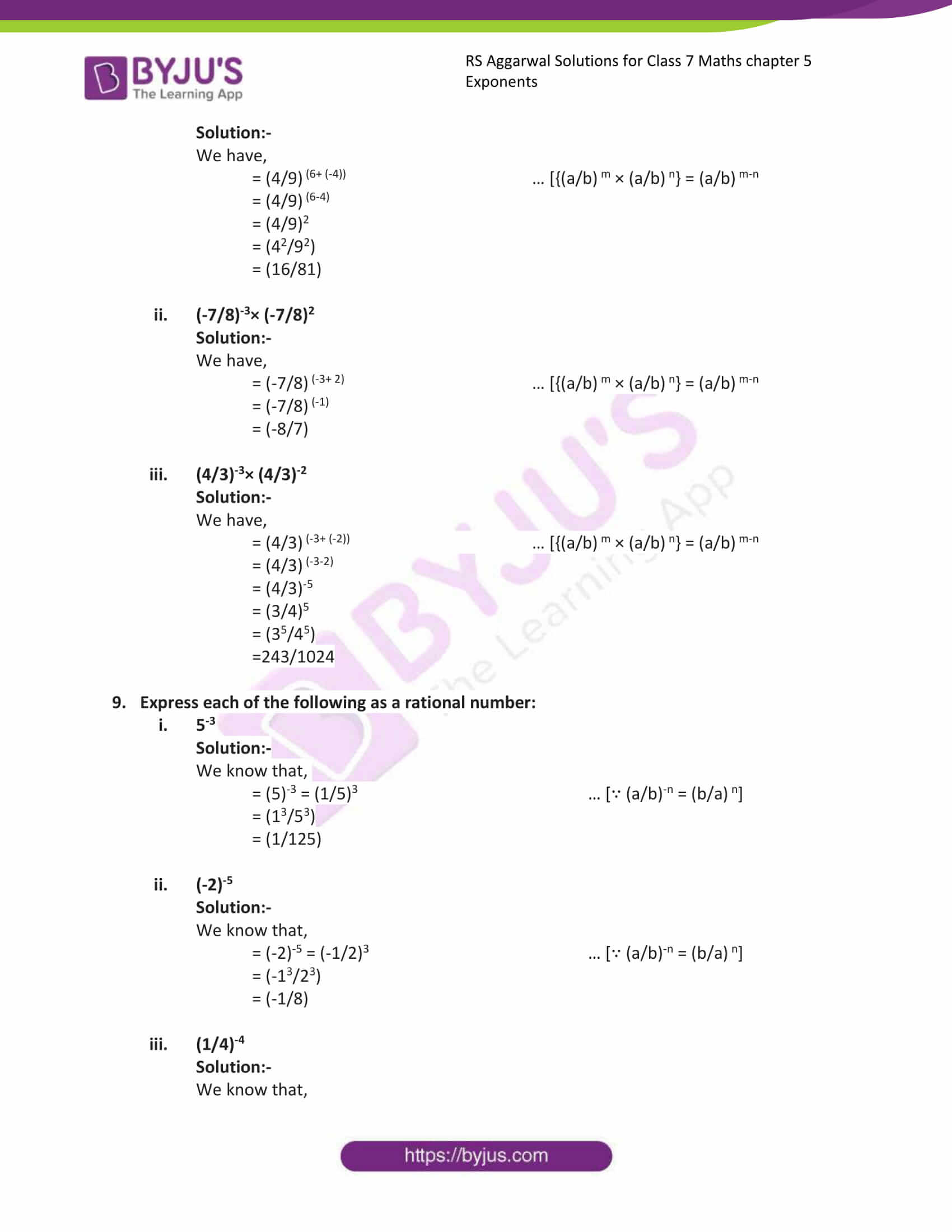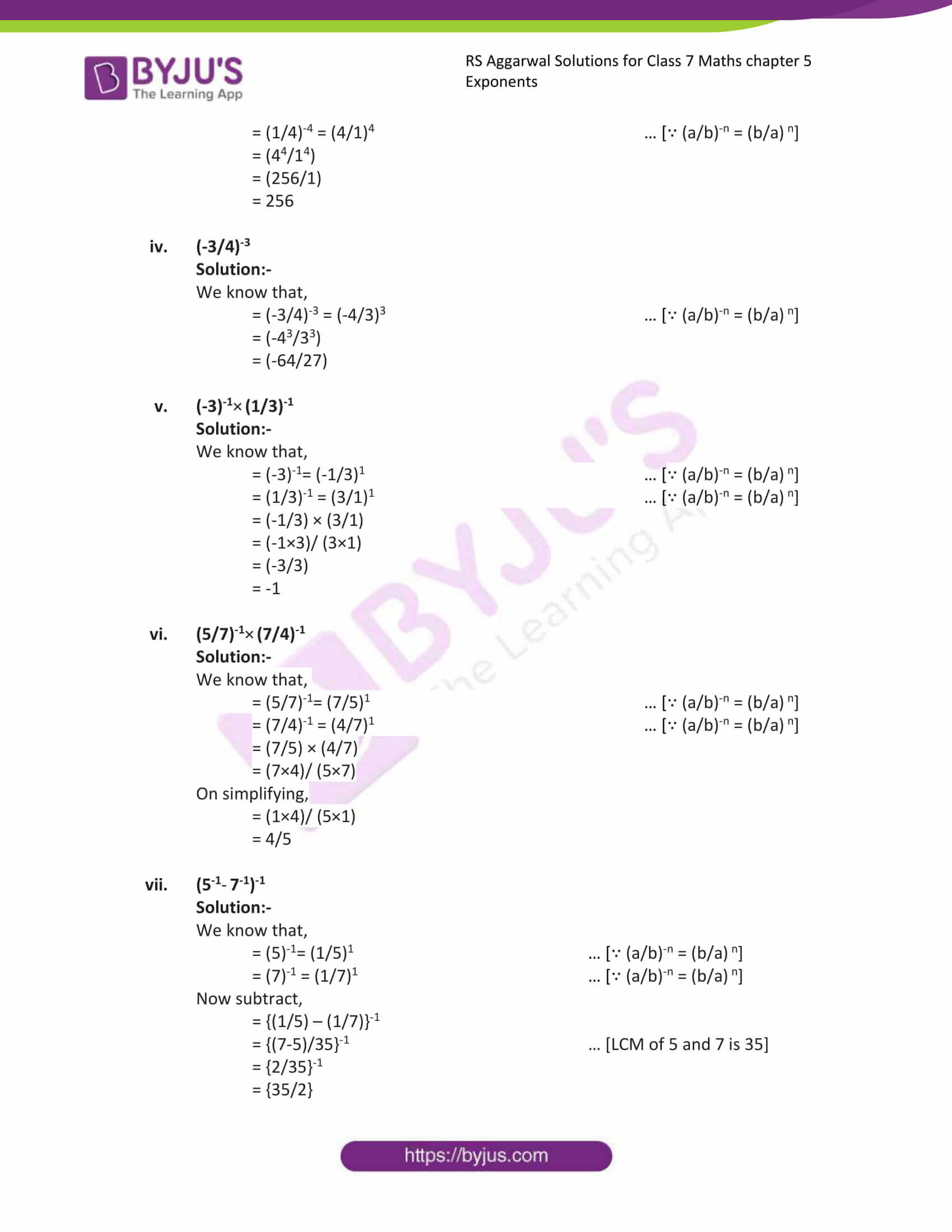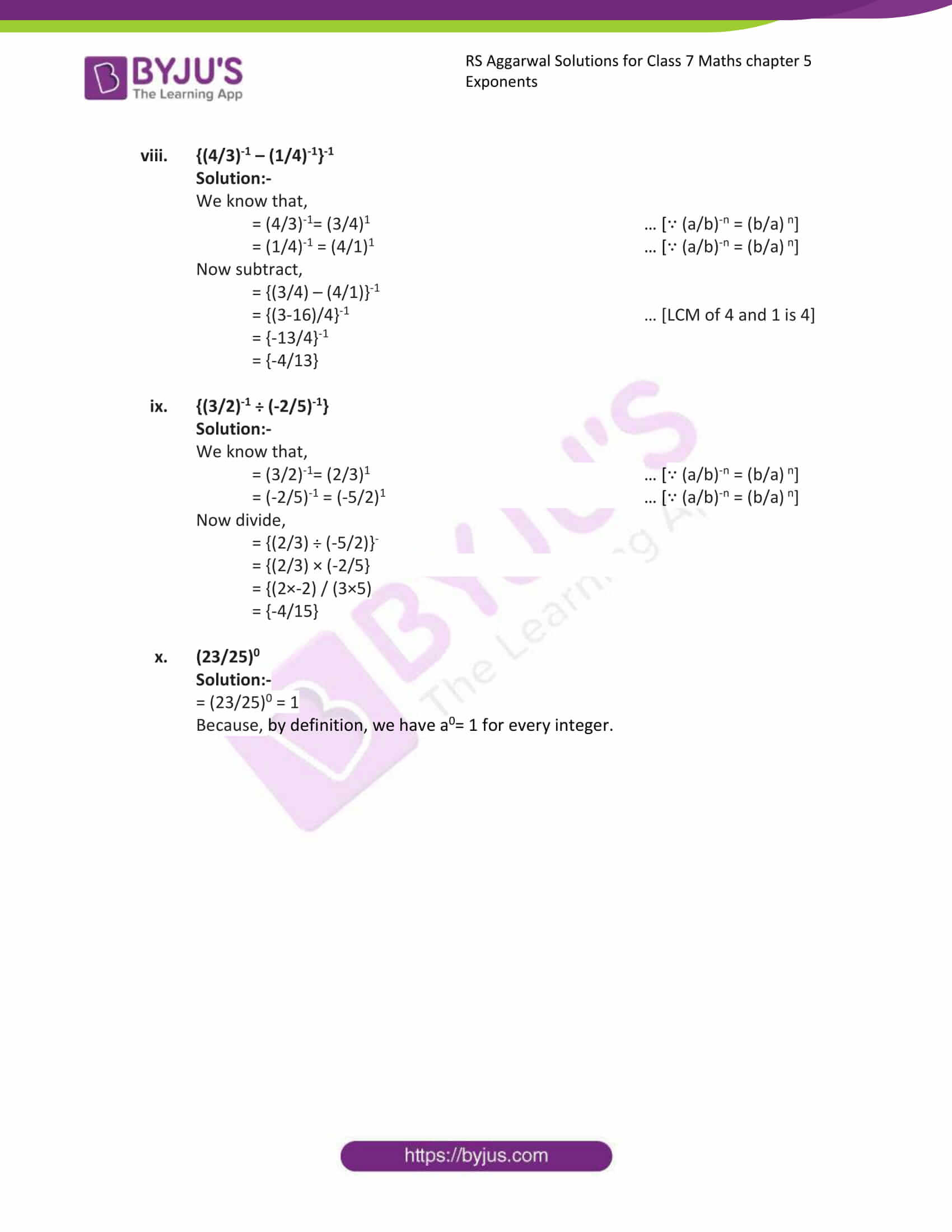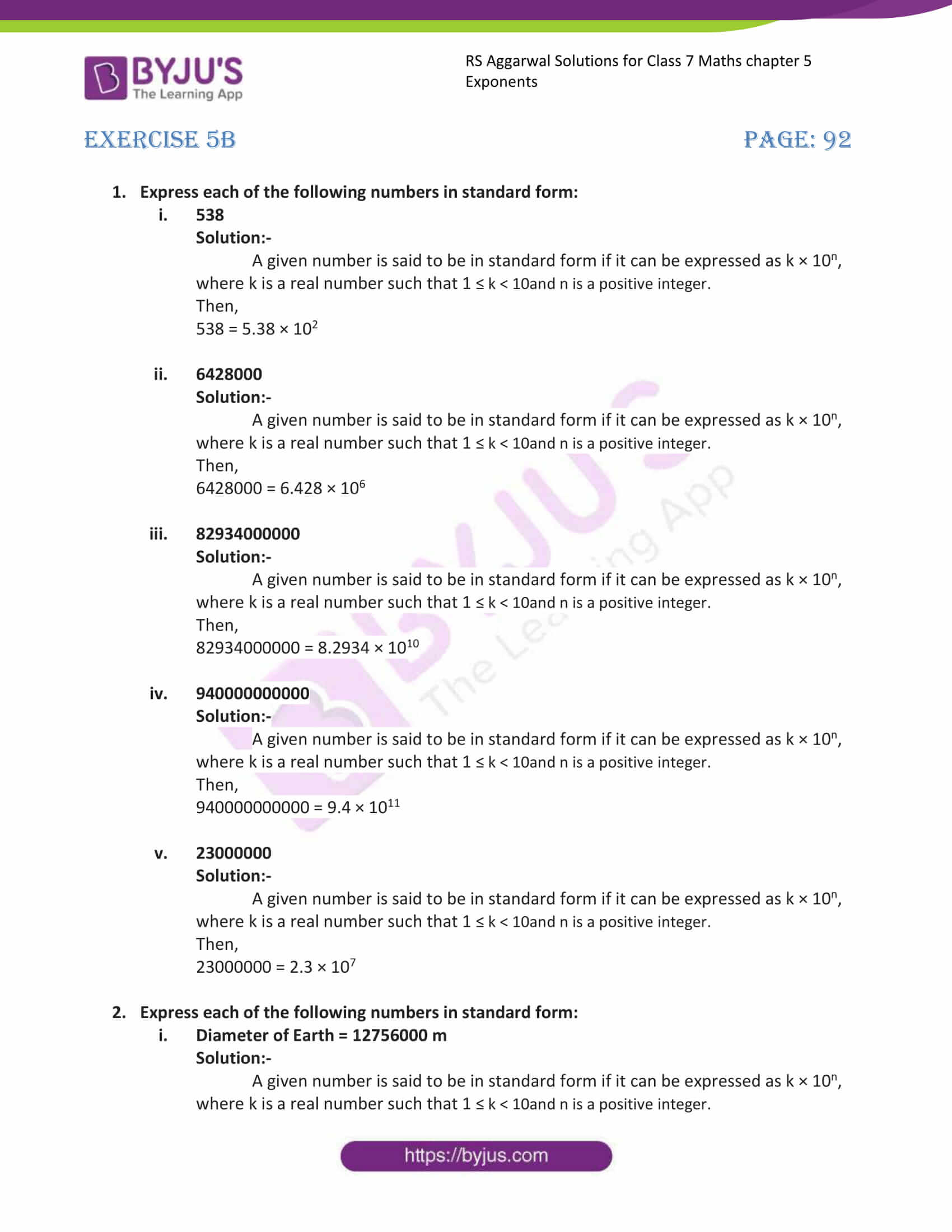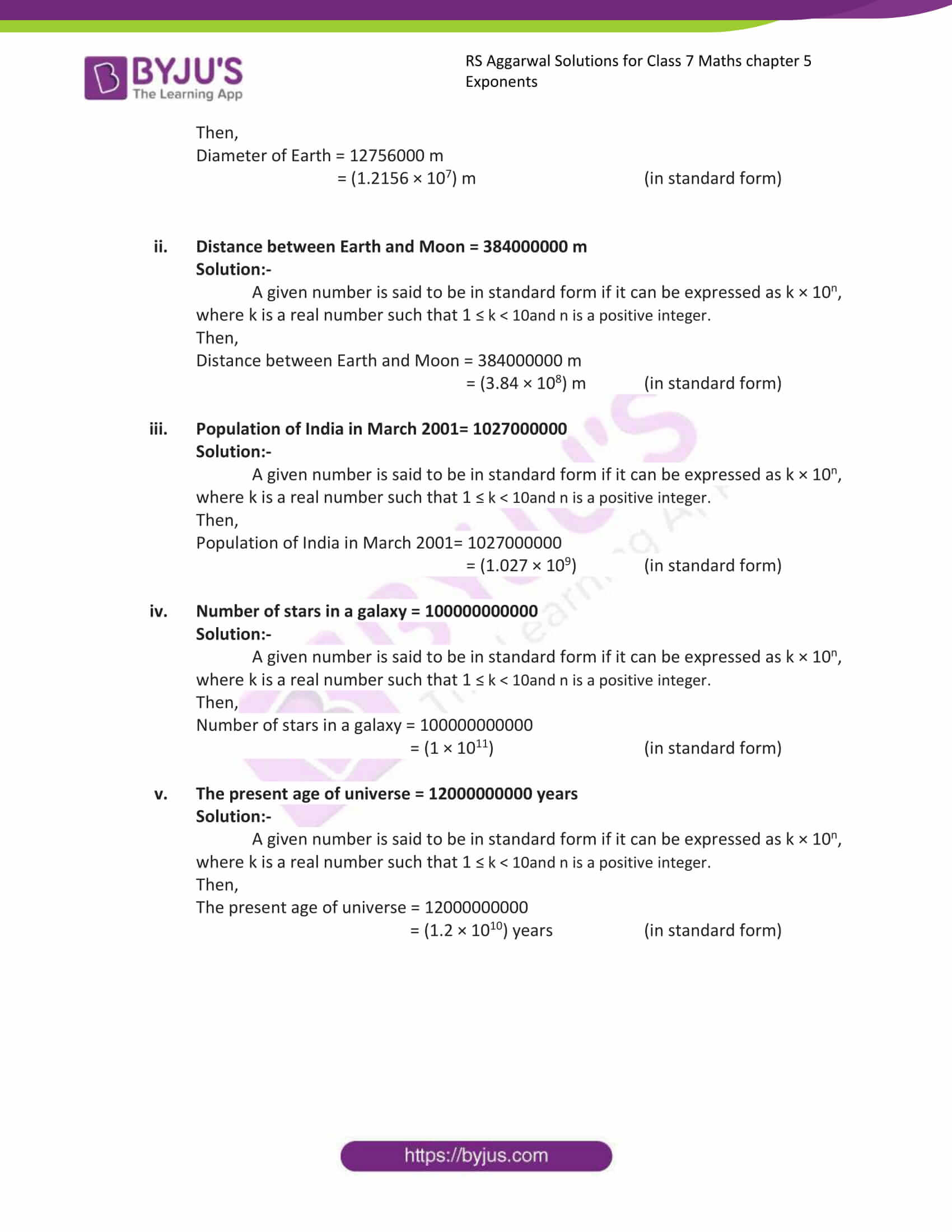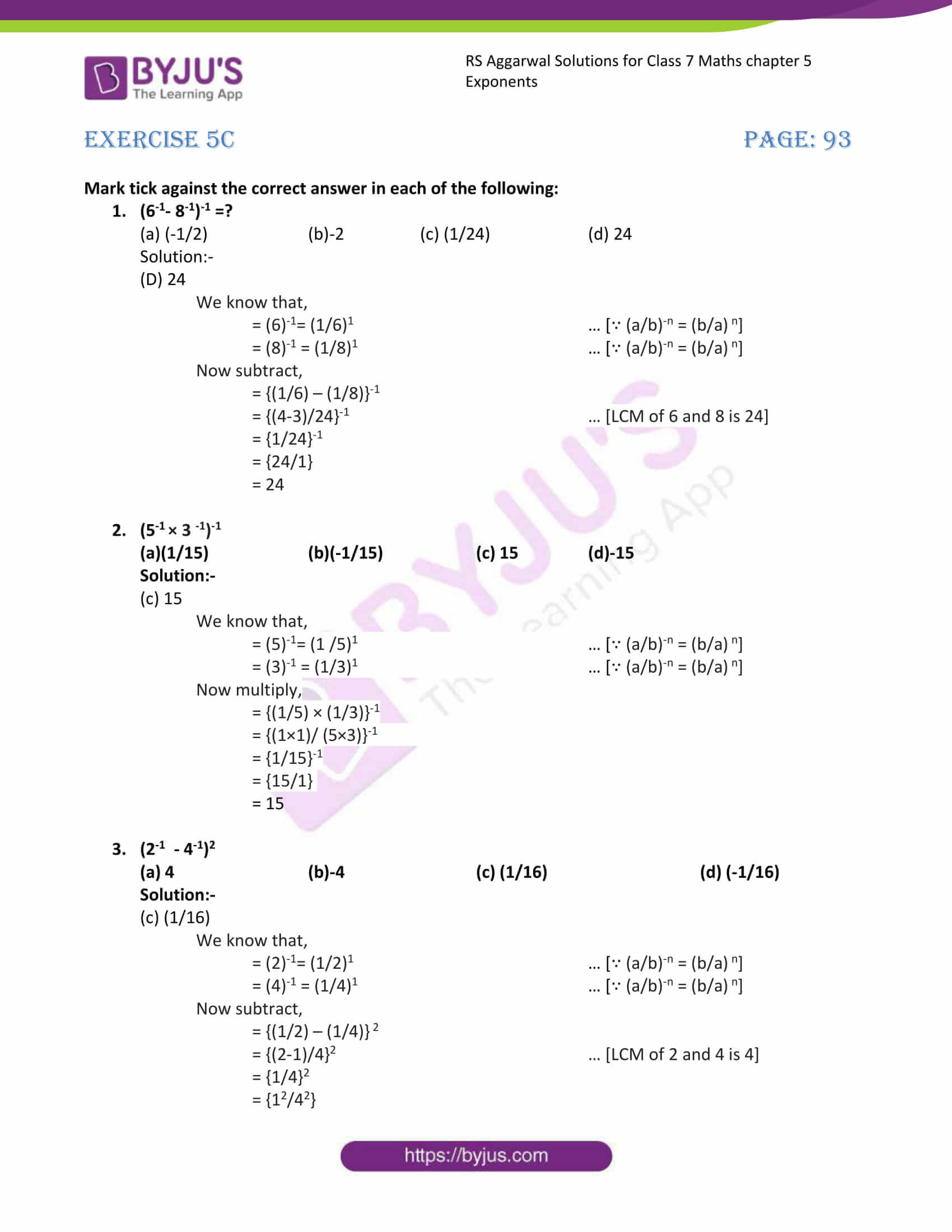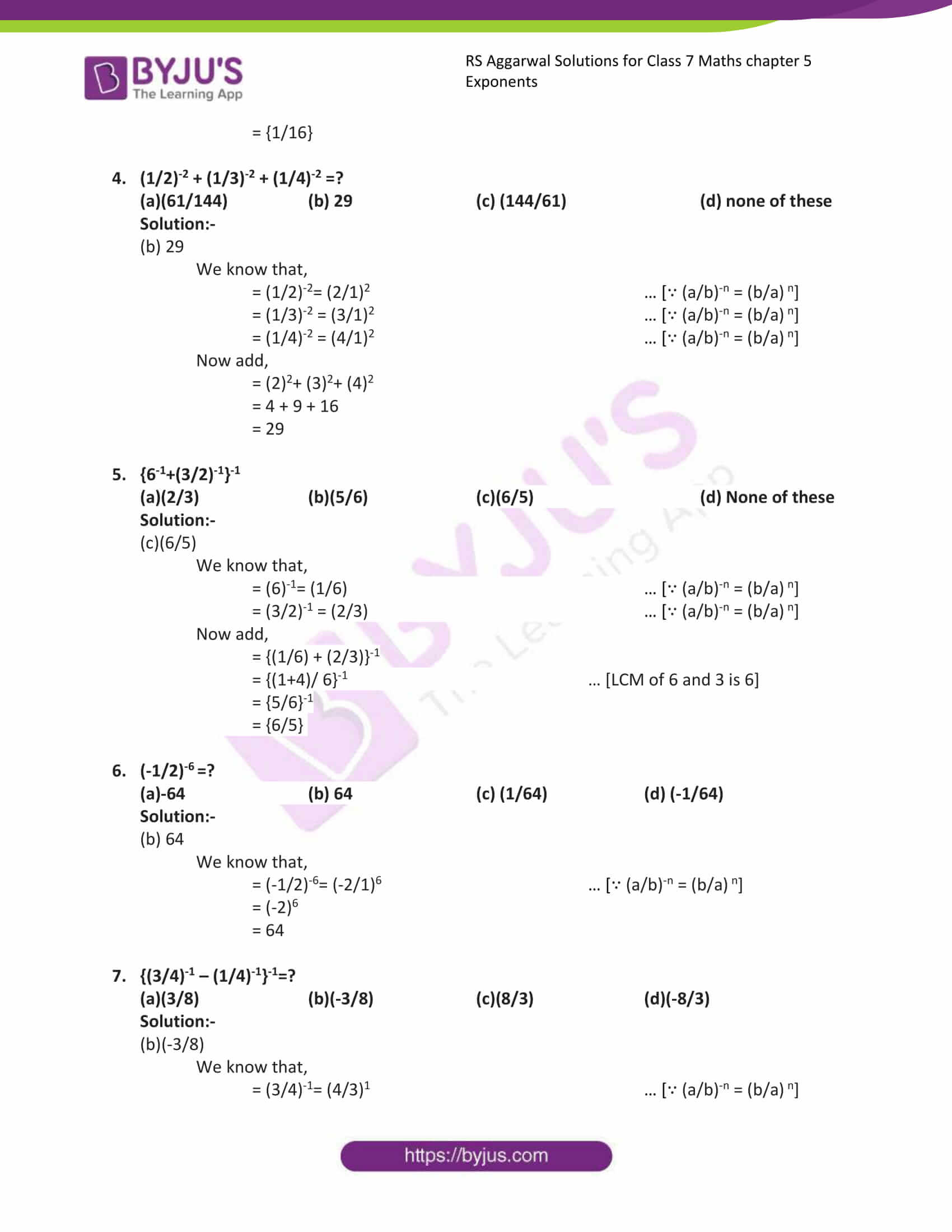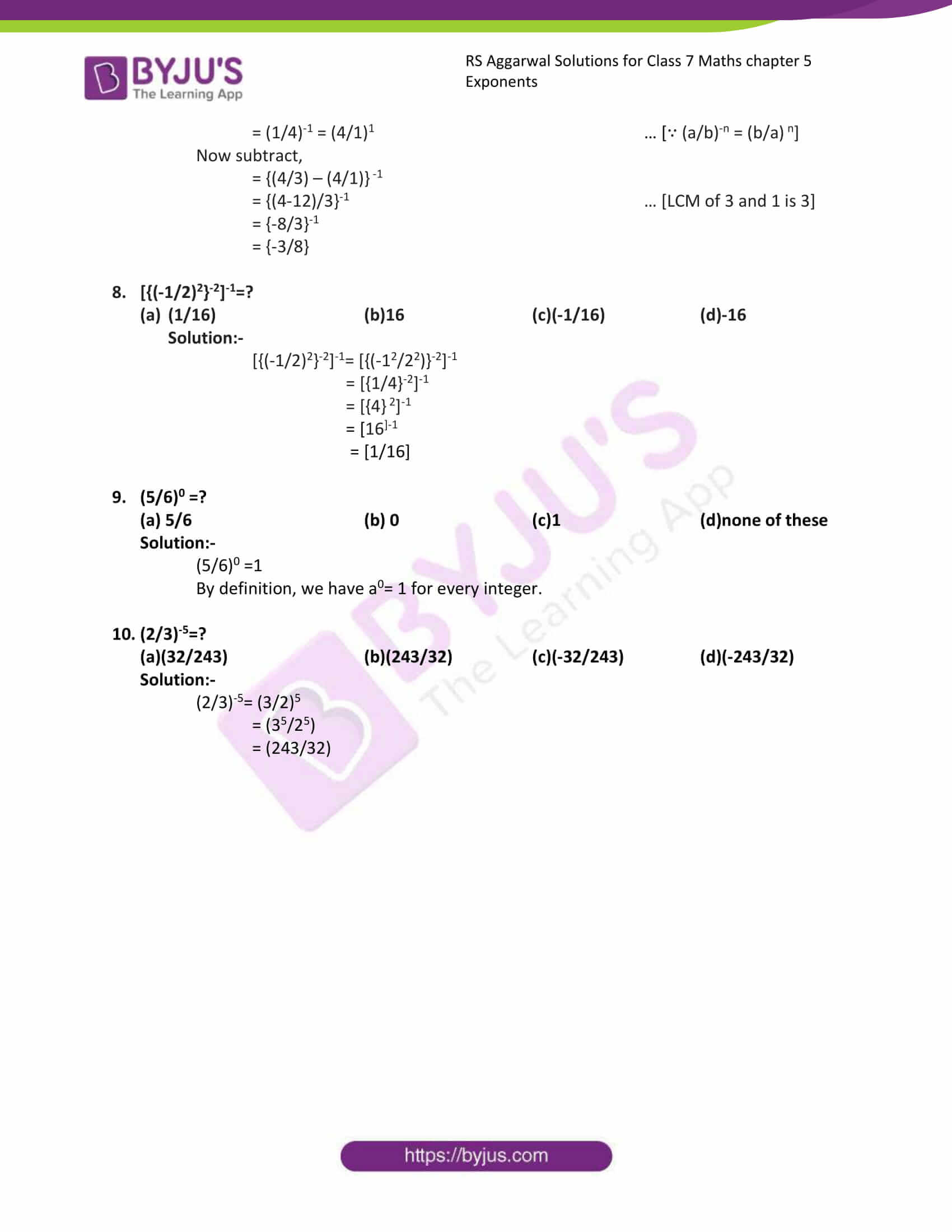Exercise 5A

Exercise 5B

Exercise 5C

## Exercise 5A

1. Write each of the following in power notation:

(i) (5/7) ×(5/7) × (5/7) × (5/7)

Solution:-

The product of rational number multiplied several times by itself can be expressed in the power notations as,

(5/7)4

(ii) (-4/3) ×(-4/3) × (-4/3) × (-4/3) × (-4/3)

Solution:-

The product of rational number multiplied several times by itself can be expressed in the power notations as,

(-4/3)5

(iii) (-1/6) ×(-1/6) × (-1/6)

Solution:-

The product of rational number multiplied several times by itself can be expressed in the power notations as,

(-1/6)3

(iv) (-8) ×(-8) × (-8) × (-8) × (-8)

Solution:-

The product of rational number multiplied several times by itself can be expressed in the power notations as,

(-8)5

2. Express each of the following in power notation:

(i) (25/36)

Solution:-

We have,

25 = 5 × 5 = (5)2

36 = 6 × 6 = (6)2

Then,

= (52/62)

∴ (5/6)2

(ii)(-27/64)

Solution:-

We have,

-27 = -3 × -3 × -3 = (-3)3

64 = 4 × 4 × 4 = (4)3

Then,

= (-33/43)

∴ (-3/4)3

(iii) (-32/243)

Solution:-

We have,

-32 = -2 × -2 × -2 × -2 × -2 = (-2)5

243 = 3 × 3 × 3 × 3 × 3 = (3)5

Then,

= (-25/35)

∴ (-2/3)5

(iv) (-1/128)

Solution:-

We have,

-1 = -1 × -1 × -1 × -1 × -1 × -1 × -1 = (-1)7

128 = 2 × 2 × 2 × 2 × 2 × 2 × 2 = (2)7

Then,

= (-17/27)

∴ (-1/2)7

3. Express each of the following a rational number:

(i) (2/3)5

Solution:-

We have,

(2/3)5 = (25/35)

= (2 × 2 × 2 × 2 × 2) / (3 × 3 × 3 × 3 × 3)

= (32/243)

(i) (-8/5)3

Solution:-

We have,

(-8/5)3 = (-83/53)

= (-8 × -8 × -8) / (5 × 5 × 5)

= (-512/125)

(ii) (-13/11)2

Solution:-

We have,

(-13/11)2 = (-132/112)

= (-13 × -13) / (11 × 11)

= (169/121)

(iii) (1/6)3

Solution:-

We have,

(1/6)3 = (13/63)

= (1 × 1 × 1) / (6 × 6 × 6)

= (1/216)

(v) (-1/2)5

Solution:-

We have,

(-1/2)5 = (-15/25)

= (-1 × -1 × -1× -1 × -1) / (2 × 2 × 2 × 2 × 2)

= (-1/32)

(vi) (-4/7)3

Solution:-

We have,

(-4/7)3 = (-43/73)

= (-4 × -4 × -4) / (7 × 7 × 7)

= (-64/343)

(vii) (-1)9

Solution:-

We have,

(-1)9 = (-19)

= (-1 × -1 × -1× -1 × -1 × -1 × -1× -1 × -1)

= (-1)

4. Express each of the following as a rational number:

(i) (4)-1

Solution:-

We have:

(4)-1 = (4/1)-1

= (1/4)1 … [∵ (a/b)-n = (b/a) n]

= (1/4)

(ii) (-6)-1

Solution:-

We have:

(-6)-1 = (-6/1)-1

= (1/-6)1 … [∵ (a/b)-n = (b/a) n]

= (-1/6)

(iii) (1/3)-1

Solution:-

We have:

(1/3)-1 = (-6/1)-1

= (3/1)1 … [∵ (a/b)-n = (b/a) n]

= 3

(iv) (–2/3)-1

Solution:-

We have:

(-2/3)-1 = (-2/3)-1

= (3/-2)1 … [∵ (a/b)-n = (b/a) n]

= (-3/2)

5. Find the reciprocal of each of the following:

(i) (3/8)4

Solution:-

We know that the reciprocal of (a/b) m is (b/a) m

Then,

Reciprocal of (3/8)4 is (8/3)4

(ii) (-5/6)11

Solution:-

We know that the reciprocal of (a/b) m is (b/a) m

Then,

Reciprocal of (-5/6)11 is (-6/5)11

(iii) (6)7

Solution:-

We know that the reciprocal of (a/b) m is (b/a) m

Then,

Reciprocal of (6)7 is (1/6)7

(iv) (-4)3

Solution:-

We know that the reciprocal of (a/b) m is (b/a) m

Then,

Reciprocal of (-4)3 is (-1/4)3

6. Find the value of each of the following:

(i) 80

Solution:-

By definition, we have a0= 1 for every integer.

∴ 80 = 1

(ii) (-3)0

Solution:-

By definition, we have a0= 1 for every integer.

∴ (-3)0 = 1

(iii) 40 + 50

Solution:-

By definition, we have a0= 1 for every integer.

∴ 40 + 50

= 1 + 1

= 2

(iv) 60 × 70

Solution:-

By definition, we have a0= 1 for every integer.

∴ 60 × 70

= 1 × 1

= 1

7. Simplify each of the following and express each as a rational number:

(i) (3/2)4 × (1/5)2

Solution:-

We have,

(34/24) = (3×3×3×3)/ (2×2×2×2) = (81/16)

(12/52) = (1×1)/ (5×5) = (1/25)

Then,

= (81/16) × (1/25)

= (81×1) / (16/25)

= (81/400)

(ii) (-2/3)5 × (-3/7)3

Solution:-

We have,

(-25/35) = (-2×-2×-2×-2×-2)/ (3×3×3×3×3) = (-32/243)

(-33/73) = (-3×-3×-3)/ (7×7×7) = (-27/343)

Then,

= (-32/243) × (-27/343)

= (-32×-27) / (243×343)

By simplifying,

= (-32×-1) / (9/343)

= (32/3087)

(iii) (-1/2)5 × 23 × (3/4)2

Solution:-

We have,

(-15/25) = (-1×-1×-1×-1×-1)/ (2×2×2×2×2) = (-1/32)

(2)3= (2×2×2) = 8

(32/42) = (3×3)/ (4×4) = (9/16)

Then,

= (-1/32) × 8 × (9/16)

= (-1×8×9) / (32× 1×16)

By simplifying,

= (-1×1×9) / (32×1×2)

= (-9/64)

(iv) (2/3)2 × (-3/5)3 × (7/2)2

Solution:-

We have,

(22/32) = (2×2)/ (3×3) = (4/9)

(-3/5)3= (-3×-3×-3) / (5×5×5) = (-27/125)

(72/22) = (7×7)/ (2×2) = (49/4)

Then,

= (4/9) × (-27/125) × (49/4)

= (4×-27×49) / (9× 125×4)

On simplifying,

= (1×-3×49) / (1×125×1)

= (-147/125)

(v) {(-3/4)3-(-5/2)3} × 42

Solution:-

We have,

= {(-33/43)-(53/23)} × 16

= {(-27/64)-(-125/8)} × 16

First we find the difference of {(-27/64)-(125/8)}

LCM of 64 and 8 is 64

= (-27×1) / (64×1) = (-27/64)

= (125×8) / (8 ×8) = (1000/64)

= (1000- (-27))/64

= (1000+27)/64

= (973/64)

= (973/64) × 16

= (973/4)

8. Simplify and express each as a rational number:

(i) (4/9)6× (4/9)-4

Solution:-

We have,

= (4/9) (6+ (-4)) … [{(a/b) m × (a/b) n} = (a/b) m-n

= (4/9) (6-4)

= (4/9)2

= (42/92)

= (16/81)

(ii) (-7/8)-3× (-7/8)2

Solution:-

We have,

= (-7/8) (-3+ 2) … [{(a/b) m × (a/b) n} = (a/b) m-n

= (-7/8) (-1)

= (-8/7)

(iii) (4/3)-3× (4/3)-2

Solution:-

We have,

= (4/3) (-3+ (-2)) … [{(a/b) m × (a/b) n} = (a/b) m-n

= (4/3) (-3-2)

= (4/3)-5

= (3/4)5

= (35/45)

=243/1024

9. Express each of the following as a rational number:

(i) 5-3

Solution:-

We know that,

= (5)-3 = (1/5)3 … [∵ (a/b)-n = (b/a) n]

= (13/53)

= (1/125)

(ii) (-2)-5

Solution:-

We know that,

= (-2)-5 = (-1/2)3 … [∵ (a/b)-n = (b/a) n]

= (-13/23)

= (-1/8)

(iii) (1/4)-4

Solution:-

We know that,

= (1/4)-4 = (4/1)4 … [∵ (a/b)-n = (b/a) n]

= (44/14)

= (256/1)

= 256

(iv) (-3/4)-3

Solution:-

We know that,

= (-3/4)-3 = (-4/3)3 … [∵ (a/b)-n = (b/a) n]

= (-43/33)

= (-64/27)

(v) (-3)-1× (1/3)-1

Solution:-

We know that,

= (-3)-1= (-1/3)1 … [∵ (a/b)-n = (b/a) n]

= (1/3)-1 = (3/1)1 … [∵ (a/b)-n = (b/a) n]

= (-1/3) × (3/1)

= (-1×3)/ (3×1)

= (-3/3)

= -1

(vi) (5/7)-1× (7/4)-1

Solution:-

We know that,

= (5/7)-1= (7/5)1 … [∵ (a/b)-n = (b/a) n]

= (7/4)-1 = (4/7)1 … [∵ (a/b)-n = (b/a) n]

= (7/5) × (4/7)

= (7×4)/ (5×7)

On simplifying,

= (1×4)/ (5×1)

= 4/5

(vii) (5-1 7-1)-1

Solution:-

We know that,

= (5)-1= (1/5)1 … [∵ (a/b)-n = (b/a) n]

= (7)-1 = (1/7)1 … [∵ (a/b)-n = (b/a) n]

Now subtract,

= {(1/5) – (1/7)}-1

= {(7-5)/35}-1 … [LCM of 5 and 7 is 35]

= {2/35}-1

= {35/2}

(viii) {(4/3)-1 – (1/4)-1}-1

Solution:-

We know that,

= (4/3)-1= (3/4)1 … [∵ (a/b)-n = (b/a) n]

= (1/4)-1 = (4/1)1 … [∵ (a/b)-n = (b/a) n]

Now subtract,

= {(3/4) – (4/1)}-1

= {(3-16)/4}-1 … [LCM of 4 and 1 is 4]

= {-13/4}-1

= {-4/13}

(ix) {(3/2)-1 ÷ (-2/5)-1}

Solution:-

We know that,

= (3/2)-1= (2/3)1 … [∵ (a/b)-n = (b/a) n]

= (-2/5)-1 = (-5/2)1 … [∵ (a/b)-n = (b/a) n]

Now divide,

= {(2/3) ÷ (-5/2)}

= {(2/3) × (-2/5}

= {(2×-2) / (3×5)

= {-4/15}

(x) (23/25)0

Solution:-

= (23/25)0 = 1

Because, by definition, we have a0= 1 for every integer.

## Exercise 5B

1. Express each of the following numbers in standard form:

(i) 538

Solution:-

A given number is said to be in standard form if it can be expressed as k × 10n, where k is a real number such that 1 ≤ k < 10and n is a positive integer.

Then,

538 = 5.38 × 102

(ii) 6428000

Solution:-

A given number is said to be in standard form if it can be expressed as k × 10n, where k is a real number such that 1 ≤ k < 10and n is a positive integer.

Then,

6428000 = 6.428 × 106

(iii) 82934000000

Solution:-

A given number is said to be in standard form if it can be expressed as k × 10n, where k is a real number such that 1 ≤ k < 10and n is a positive integer.

Then,

82934000000 = 8.2934 × 1010

(iv) 940000000000

Solution:-

A given number is said to be in standard form if it can be expressed as k × 10n, where k is a real number such that 1 ≤ k < 10and n is a positive integer.

Then,

940000000000 = 9.4 × 1011

(v) 23000000

Solution:-

A given number is said to be in standard form if it can be expressed as k × 10n, where k is a real number such that 1 ≤ k < 10and n is a positive integer.

Then,

23000000 = 2.3 × 107

2. Express each of the following numbers in standard form:

(i) Diameter of Earth = 12756000 m

Solution:-

A given number is said to be in standard form if it can be expressed as k × 10n, where k is a real number such that 1 ≤ k < 10and n is a positive integer.

Then,

Diameter of Earth = 12756000 m

= (1.2156 × 107) m (in standard form)

(ii) Distance between Earth and Moon = 384000000 m

Solution:-

A given number is said to be in standard form if it can be expressed as k × 10n, where k is a real number such that 1 ≤ k < 10and n is a positive integer.

Then,

Distance between Earth and Moon = 384000000 m

= (3.84 × 108) m (in standard form)

(iii) Population of India in March 2001= 1027000000

Solution:-

A given number is said to be in standard form if it can be expressed as k × 10n, where k is a real number such that 1 ≤ k < 10and n is a positive integer.

Then,

Population of India in March 2001= 1027000000

= (1.027 × 109) (in standard form)

(iv) Number of stars in a galaxy = 100000000000

Solution:-

A given number is said to be in standard form if it can be expressed as k × 10n, where k is a real number such that 1 ≤ k < 10and n is a positive integer.

Then,

Number of stars in a galaxy = 100000000000

= (1 × 1011) (in standard form)

(v) The present age of universe = 12000000000 years

Solution:-

A given number is said to be in standard form if it can be expressed as k × 10n, where k is a real number such that 1 ≤ k < 10and n is a positive integer.

Then,

The present age of universe = 12000000000

= (1.2 × 1010) years (in standard form)

## Exercise 5C

Mark tick against the correct answer in each of the following:

1. (6-1– 8-1)-1 =?

(a) (-1/2) (b)-2 (c) (1/24) (d) 24

Solution:-

(D) 24

We know that,

= (6)-1= (1/6)1 … [∵ (a/b)-n = (b/a) n]

= (8)-1 = (1/8)1 … [∵ (a/b)-n = (b/a) n]

• Now subtract,
• = {(1/6) – (1/8)}-1

= {(4-3)/24}-1 … [LCM of 6 and 8 is 24]

= {1/24}-1

= {24/1}

= 24

2. (5-1 × 3 -1)-1

(a)(1/15) (b)(-1/15) (c) 15 (d)-15

Solution:-

(c) 15

We know that,

= (5)-1= (1 /5)1 … [∵ (a/b)-n = (b/a) n]

= (3)-1 = (1/3)1 … [∵ (a/b)-n = (b/a) n]

Now multiply,

= {(1/5) × (1/3)}-1

= {(1×1)/ (5×3)}-1

= {1/15}-1

= {15/1}

= 15

3. (2-1 – 4-1)2

(a) 4 (b)-4 (c) (1/16) (d) (-1/16)

Solution:-

(c) (1/16)

We know that,

= (2)-1= (1/2)1 … [∵ (a/b)-n = (b/a) n]

= (4)-1 = (1/4)1 … [∵ (a/b)-n = (b/a) n]

Now subtract,

= {(1/2) – (1/4)} 2

= {(2-1)/4}2 … [LCM of 2 and 4 is 4]

= {1/4}2

= {12/42}

= {1/16}

4. (1/2)-2 + (1/3)-2 + (1/4)-2 =?

(a)(61/144) (b) 29 (c) (144/61) (d) none of these

Solution:-

(b) 29

We know that,

= (1/2)-2= (2/1)2 … [∵ (a/b)-n = (b/a) n]

= (1/3)-2 = (3/1)2 … [∵ (a/b)-n = (b/a) n]

= (1/4)-2 = (4/1)2 … [∵ (a/b)-n = (b/a) n]

= (2)2+ (3)2+ (4)2

= 4 + 9 + 16

= 29

5. {6-1+(3/2)-1}-1

(a)(2/3) (b)(5/6) (c)(6/5) (d) None of these

Solution:-

(c)(6/5)

We know that,

= (6)-1= (1/6) … [∵ (a/b)-n = (b/a) n]

= (3/2)-1 = (2/3) … [∵ (a/b)-n = (b/a) n]

= {(1/6) + (2/3)}-1

= {(1+4)/ 6}-1 … [LCM of 6 and 3 is 6]

= {5/6}-1

= {6/5}

6. (-1/2)-6 =?

(a)-64 (b) 64 (c) (1/64) (d) (-1/64)

Solution:-

(b) 64

We know that,

= (-1/2)-6= (-2/1)6 … [∵ (a/b)-n = (b/a) n]

= (-2)6

= 64

7. {(3/4)-1 – (1/4)-1}-1=?

(a)(3/8) (b)(-3/8) (c)(8/3) (d)(-8/3)

Solution:-

(b)(-3/8)

We know that,

= (3/4)-1= (4/3)1 … [∵ (a/b)-n = (b/a) n]

= (1/4)-1 = (4/1)1 … [∵ (a/b)-n = (b/a) n]

Now subtract,

= {(4/3) – (4/1)} -1

= {(4-12)/3}-1 … [LCM of 3 and 1 is 3]

= {-8/3}-1

= {-3/8}

8. [{(-1/2)2}-2]-1=?

(1/16) (b)16 (c)(-1/16) (d)-16

Solution:-

[{(-1/2)2}-2]-1= [{(-12/22)}-2]-1

= [{1/4}-2]-1

= [{4} 2]-1

= -1

= [1/16]

9. (5/6)0 =?

(a) 5/6 (b) 0 (c)1 (d)none of these

Solution:-

(5/6)0 =1

By definition, we have a0= 1 for every integer.

10. (2/3)-5=?

(a)(32/243) (b)(243/32) (c)(-32/243) (d)(-243/32)

Solution:-

(2/3)-5= (3/2)5

= (35/25)

= (243/32)

## RS Aggarwal Solutions for Class 7 Maths Chapter 5 – Exponents

Chapter 5 – Exponents contain 3 exercises and the RS Aggarwal Solutions available on this page provide solutions to the questions present in the exercises. Now, let us have a look at some of the essential concepts discussed in this chapter.

• Laws of Exponents
• Expressing Large Numbers in Standard Form
• Numbers in Expanded Form
• Square Root

### Chapter Brief of RS Aggarwal Solutions for Class 7 Maths Chapter 5 – Exponents

RS Aggarwal Solutions for Class 7 Maths Chapter 5 – Exponents. When a number is multiplied by itself frequently, then we raise it to power. The power in the exponent expresses the number of times that we want to carry out the multiplication process. In this chapter, we will learn about powers, exponents and their peripheral rules. The solutions are solved in such a way that students will understand clearly, and they will never get confused.